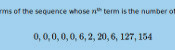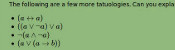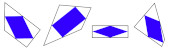mscroggs.co.uk
mscroggs.co.uksubscribe

# Blog

## Interesting tautologies

2020-05-03
This is a post I wrote for The Aperiodical's Big Lock-Down Math-Off. You can vote for (or against) me here until 9am on Tuesday...
A few years ago, I made @mathslogicbot, a Twitter bot that tweets logical tautologies.
The statements that @mathslogicbot tweets are made up of variables (a to z) that can be either true or false, and the logical symbols $$\lnot$$ (not), $$\land$$ (and), $$\lor$$ (or), $$\rightarrow$$ (implies), and $$\leftrightarrow$$ (if and only if), as well as brackets. A tautology is a statement that is always true, whatever values are assigned to the variables involved.
To get an idea of how to interpret @mathslogicbot's statements, let's have a look at a few tautologies:
$$( a \rightarrow a )$$. This says "a implies a", or in other words "if a is true, then a is true". Hopefully everyone agrees that this is an always-true statement.
$$( a \lor \lnot a )$$. This says "a or not a": either a is true, or a is not true
$$(a\leftrightarrow a)$$. This says "a if and only if a".
$$\lnot ( a \land \lnot a )$$. This says "not (a and not a)": a and not a cannot both be true.
$$( \lnot a \lor \lnot \lnot a )$$. I'll leave you to think about what this one means.
(Of course, not all statements are tautologies. The statement $$(b\land a)$$, for example, is not a tautology as is can be true or false depending on the values of $$a$$ and $$b$$.)
While looking through @mathslogicbot's tweets, I noticed that a few of them are interesting, but most are downright rubbish. This got me thinking: could I get rid of the bad tautologies like these, and make a list of just the "interesting" tautologies. To do this, we first need to think of different ways tautologies can be bad.
Looking at tautologies the @mathslogicbot has tweeted, I decided to exclude:
• tautologies like $$(a\rightarrow\lnot\lnot\lnot\lnot a)$$ that contain more than one $$\lnot$$ in a row.
• tautologies like $$((a\lor\lnot a)\lor b)$$ that contain a shorter tautology. Instead, tautologies like $$(\text{True}\lor b)$$ should be considered.
• tautologies like $$((a\land\lnot a)\rightarrow b)$$ that contain a shorter contradiction (the opposite of a tautology). Instead, tautologies like $$(\text{False}\rightarrow b)$$ should be considered.
• tautologies like $$(\text{True}\lor\lnot\text{True})$$ or $$((b\land a)\lor\lnot(b\land a)$$ that are another tautology (in this case $$(a\lor\lnot a)$$) with a variable replaced with something else.
• tautologies containing substatements like $$(a\land a)$$, $$(a\lor a)$$ or $$(\text{True}\land a)$$ that are equivalent to just writing $$a$$.
• tautologies that contain a $$\rightarrow$$ that could be replaced with a $$\leftrightarrow$$, because it's more interesting if the implication goes both ways.
• tautologies containing substatements like $$(\lnot a\lor\lnot b)$$ or $$(\lnot a\land\lnot b)$$ that could be replaced with similar terms (in these cases $$(a\land b)$$ and $$(a\lor b)$$ respectively) without the $$\lnot$$s.
• tautologies that are repeats of each other with the order changed. For example, only one of $$(a\lor\lnot a)$$ and $$(\lnot a\lor a)$$ should be included.
After removing tautologies like these, some of my favourite tautologies are:
• $$( \text{False} \rightarrow a )$$
• $$( a \rightarrow ( b \rightarrow a ) )$$
• $$( ( \lnot a \rightarrow a ) \leftrightarrow a )$$
• $$( ( ( a \leftrightarrow b ) \land a ) \rightarrow b )$$
• $$( ( ( a \rightarrow b ) \leftrightarrow a ) \rightarrow a )$$
• $$( ( a \lor b ) \lor ( a \leftrightarrow b ) )$$
• $$( \lnot ( ( a \land b ) \leftrightarrow a ) \rightarrow a )$$
• $$( ( \lnot a \rightarrow b ) \leftrightarrow ( \lnot b \rightarrow a ) )$$
You can find a list of the first 500 "interesting" tautologues here. Let me know on Twitter which is your favourite. Or let me know which ones you think are rubbish, and we can further refine the list...

### Similar postsLogical contradictionsLogic bot, pt. 2Logic botA surprising fact about quadrilaterals

Comments in green were written by me. Comments in blue were not written by me.